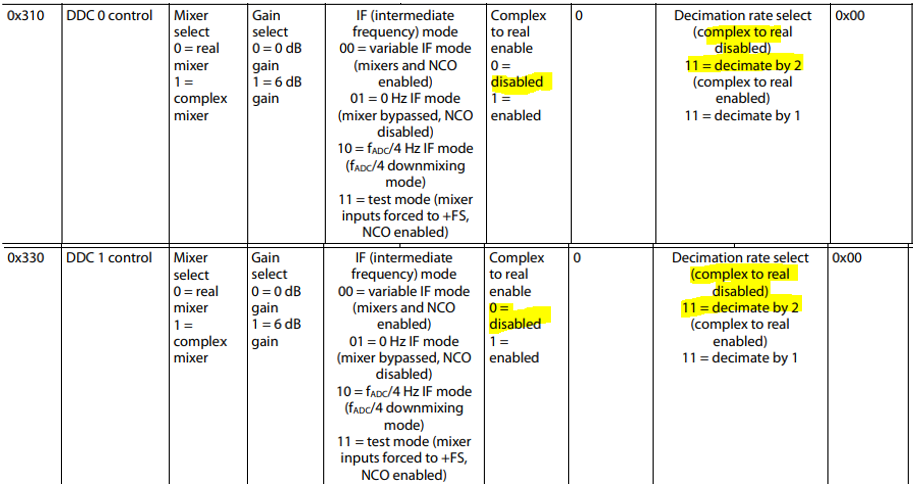Post Go back to editing

Category: Datasheet/Specs

1.  How exactly do I set the decimation ratio? Is it a setting in SPIController? Also, can I set it to any integer value, or does it have to be a power of 2?
2.  What are the “values” of the ADC? That is, when I acquire samples in VisualAnalog, are the sample values in Volts? From the AD9234 datasheet I know the reference voltage is 1V, so are the sample values just relative to the reference voltage, meaning they are in Volts?

Thank you,

Shawn

## Top Replies

Parents
• Hi s6sheng,

1. How exactly do I set the decimation ratio? Is it a setting in SPIController? Also, can I set it to any integer value, or does it have to be a power of 2?

- You can set the decimation ratio using SPIController by writing in registers 0x200, and 0x201 (on ADCBase2 in SPIController). The control registers for the DDCs are 0x310 and 0x330 (on ADCBase3 in SPIController). You cannot set the decimation ratio to any integer value; can only set it to 'decimate by 2'. If you want to use DDCs with wide decimation options, you can consider using AD9680 since the AD9680 is the source board of AD9234. Kindly try the example provided in page 45 of the datasheet.2.  What are the “values” of the ADC? That is, when I acquire samples in VisualAnalog, are the sample values in Volts? From the AD9234 datasheet I know the reference voltage is 1V, so are the sample values just relative to the reference voltage, meaning they are in Volts?

- Do you refer the 'values' as the digital output of the ADC? You can refer to the reconstructed analog signal and hex equivalent on the VA canvas attached in the figure below . The reconstructed analog signal is based on the input voltage range and the sample data length. You can set the data output format in the Output Formatter.Regards,

Xavier

• Hi Xavier,

Thank you for your reply. I will try setting the decimation ratio in SPIController as you suggested.

For the values, I meant the reconstructed analog signal values. Are these analog signal values that are output to CSV voltages? How can I calculate the actual voltage value of the input signal from these reconstructed analog signal values?

Thank you,

Shawn

• Hi s6sheng,

Yes, the CSV file is the compiled values of the reconstructed analog signal values.  The calculation of the voltage value is dependent on the peak-to-peak differential input signal of the ADC. You can refer to this tutorial note MT-009: Data Converter Codes-Can You Decode Them? (analog.com) specifically on the bipolar codes since AD9234 uses twos complement data format.

Regards,

Xavier

• I see, thank you. Just to clarify, the peak-to-peak differential input range for the AD9234-500 is 1.63Vpp (from the datasheet)? This corresponds to the Full Scale correct?

And a follow up question regarding SPIController, I do not see registers 0x200 and 0x201 in ADCBase2 (see below).Also, for setting the DDC Decimation Ratio: since there are 4 DDC control registers, if I wanted to have an overall decimation ratio of 2, I would set each control register to "Complex (I/Q) Decimate by 8"?Thank you,

Shawn

• I see, thank you. Just to clarify, the peak-to-peak differential input range for the AD9234-500 is 1.63Vpp (from the datasheet)? This corresponds to the Full Scale correct?

And a follow up question regarding SPIController, I do not see registers 0x200 and 0x201 in ADCBase2 (see below).Also, for setting the DDC Decimation Ratio: since there are 4 DDC control registers, if I wanted to have an overall decimation ratio of 2, I would set each control register to "Complex (I/Q) Decimate by 8"?Thank you,

Shawn

Children
• Hi Shawn,

Yes, the typical peak-to-peak differential voltage for AD9234-500EBZ is 1.63Vpp and also the FS, but as for bipolar codes it should be translated as +/- (1.63Vpp/2) corresponding to +0.815Vp to -0.815Vp as +FS to -FS.

I stand corrected with regards to registers 0x200 and 0x201, that should be in ADCBase0 as depicted in the figure below.As for DDC, AD9234 is limited to DDC0 and DDC1. If you want to set to decimate to 2, it should be consistent with the DDC as per registers below (Complex I/Q decimate by 2).Regards,

Xavier